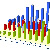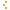MSTE
Office for Mathematics, Science, and Technology Education
College of Education @ University of Illinois

# MSTE Online Resource CatalogData Analysis and Probability

«Show all resources»

«Back to Categories»

Sort By: Title Date

### InteractiveBest FitBuffon's NeedleChi-Squared Analysis of MarblesCliff Hanger Title Brief Description Category Date Generate the best fit of a line. Data Analysis and Probability 6/10/2014 Estimate Pi by calculating the geometrical probability of a needle landing on a line. Data Analysis and Probability 5/28/2014 Find the Chi-squared value of drawing a number of random marbles. Data Analysis and Probability 12/8/1999 Ask Nick to take a random step while he braves the edge of a cliff. Data Analysis and Probability 11/3/2005 Learn about probability and hidden states with the MiSTEam Data Analysis and Probability 11/14/2005 Remove your chips with rolls of the dice. Data Analysis and Probability 9/19/2002 A Game that introduces probability from Hands-on to Resampling Stats Data Analysis and Probability 7/16/1997 Track the spread of a disease among hermits using this interactive Java applet Data Analysis and Probability 6/15/2000 Plot points on a graph and then fit the data with a polynomial of a chosen degree. Data Analysis and Probability 4/8/2001 Pick your favorite door and play the game of chance with Monty. Data Analysis and Probability 9/23/2003 Race cars by rolling a die. Data Analysis and Probability 1/28/2002 Find out just how likely it is that two or more random Birthdays are found on the same day. Data Analysis and Probability 9/9/2016 Launch a rocket if it passes all stages of its flight tests. Data Analysis and Probability 8/8/1999 A virtual scratch card with user-defined properties. Data Analysis and Probability 4/20/2005 This page offers statistical programs that can be downloaded directly into the TI-82 or 83. Data Analysis and Probability 2/2/1999 Toss these digital dice and see what numbers roll up. Data Analysis and Probability 6/16/1998

### Lessons

Title Brief Description Category Date
A two day unit plan that incorporates decision-making ideas from statistics into the science classroom. Data Analysis and Probability
10/21/1997
Lesson to introduce students both to Mathematica and to the Monte Carlo method of solving probability problems Data Analysis and Probability
10/21/1997
Use the stock market to calculate commissions and costs for buying and selling stock. Data Analysis and Probability
10/2/1995
Understand statistical terms such as expectation, significance, and chi-square Data Analysis and Probability
4/5/1996
Introduction to measure of central tendency and variation. Data Analysis and Probability
4/5/1996
Your task is to match up the correlation coefficients with the plots. Data Analysis and Probability
2/21/1996
This lesson tracks the spread of a disease on a desert island inhabited by hermits. Data Analysis and Probability
5/6/1996
Introduction to Descriptive Statistics with Mode, Median, Mean, and Range Data Analysis and Probability
7/16/1997
Student activities to explore the concept of expected value Data Analysis and Probability
5/6/1996
This web page contains nine lessons that use the TI-92 and nine programs for the TI-92. Data Analysis and Probability
7/20/1997